# KSEEB Solutions for Class 9 Maths Chapter 5 Triangles Ex 5.5

In this chapter, we provide KSEEB SSLC Class 9 Maths Chapter 5 Triangles Ex 5.5 for English medium students, Which will very helpful for every student in their exams. Students can download the latest KSEEB SSLC Class 9 Maths Chapter 5 Triangles Ex 5.5 pdf, free KSEEB SSLC Class 9 Maths Chapter 5 Triangles Ex 5.5 pdf download. Now you will get step by step solution to each question.

## Karnataka Board Class 9 Maths Chapter 5 Triangles Ex 5.5

Question 1.
ABC is a triangle. Locate a point in the interior of ∆ABC which is equidistant from all the vertices of ∆ ABC.
Solution:
ABC is a triangle. To locate a point in the interior of ∆ABC which is equidistant from all the vertices of ∆ABC we have to find out circumcentre means the point where three perpendicular bisectors meet.
E.g. Three sides of ∆ABC are,
AB = 5 cm, BC = 4 cm, and AC = 6 cm.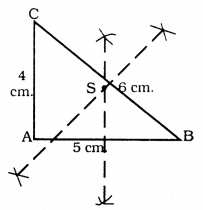‘S’ is the circumcentre of ∆ABC

Question 2.
In a triangle locate a point in its interior which is equidistant from all the sides of the triangle.
Solution:
Incentre is equidistant from three sides of a ∆. This is at the point where angular bisectors meet. This is called T.
E.g. In ∆ABC, AB = 6 cm, ∠B = 100°, ∠A = 50°.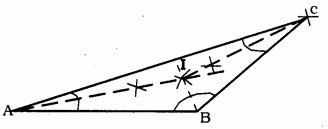Point T is equidistant from three sides.

Question 3.
In a huge park, people are concentrated at three points
A: where there are different slides and swings for children,
B: near which a man-made lake is situated,
C: which is near to a large parking and exit.
Where should an icecream parlour be set up so that the maximum number of persons can approach it?
(Hint: The parlour should be equidistant from A, B, and C).
Solution:
A: In a park where there are different slides and swings for children.
B: near which a man-made lake is situated.
C: which is near to a large parking and exit.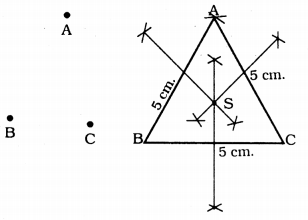In ∆ABC, to locate a point equidistant from three sides, we have to find out perpendicular bisectors, which means where all perpendicular bisectors meet. This is called S’.
AB = BC = CA = 5 cm.
∴ The ice cream shop is at ‘S’.

Question 4.
Complete the hexagonal and star-shaped Rangolies by filling them with as many equilateral triangles of side 1 cm as you can. Count the number of triangles in each case. Which has more triangles?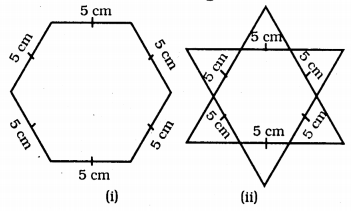Solution:
Fig. (i): Regular Hexagonal with side 5 cm. is constructed. It has 6 equal sides.
Measure of each side is 5 cm.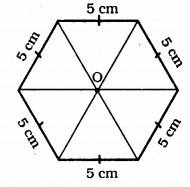Number of equilateral ∆ with 1 cm side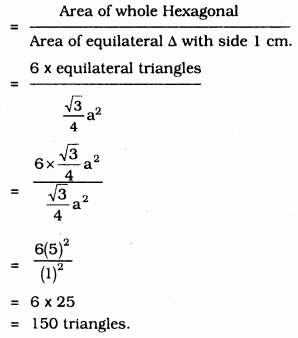In Fig. (ii) : there are 12 equilateral triangles with side 5 cm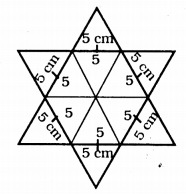∴ Number of equilateral A with 1 cm side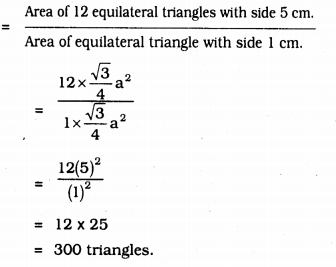All Chapter KSEEB Solutions For Class 9 Maths

—————————————————————————–

All Subject KSEEB Solutions For Class 9

*************************************************

I think you got complete solutions for this chapter. If You have any queries regarding this chapter, please comment on the below section our subject teacher will answer you. We tried our best to give complete solutions so you got good marks in your exam.

If these solutions have helped you, you can also share kseebsolutionsfor.com to your friends.

Best of Luck!!